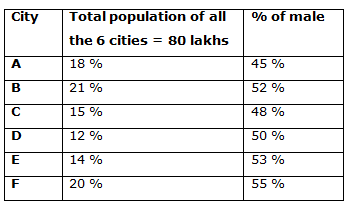# IBPS Clerk Prelims 2018 – Quantitative Aptitude Questions Day- 53

Dear Readers, Bank Exam Race for the Year 2018 is already started, To enrich your preparation here we have providing new series of Practice Questions on Quantitative Aptitude – Section. Candidates those who are preparing for IBPS Clerk Prelims 2018 Exams can practice these questions daily and make your preparation effective.

[WpProQuiz 4596]

1) P, Q and R started a business by investing Rs. 45000, 58000 and 64000 respectively. After one year, P withdraws Rs. 5000 and Q invested Rs. 7000 more. And after another one more year, R withdraws Rs. 14000. If the total profit at the end of 3 year is Rs. 265140, then find the share of Q?

a) Rs. 95640

b) Rs. 101520

c) Rs. 86180

d) Rs. 113270

e) None of these

2) An aero plane which flies at the speed of 676 km/hr in 6 hours. To cover the same distance in 4 hours, it would flies at the speed of?

a) 1014 Km/hr

b) 986 Km/hr

c) 828 Km/hr

d) 1120 Km/hr

e) None of these

3) 250 m long train running at the speed of 50 Km/hr. Find the time taken by the train to cross a man standing on the platform of length 300 m?

a) 24 (2/5) sec

b) 22 (1/5) sec

c) 26 (4/5) sec

d) 39 (3/5) sec

e) None of these

4) 5 years ago, the ratio of ages of Raghav and Shyam is 5: 4. The ratio of ages of Raghav 3 years after and that of Shyam 4 years ago is 11: 7. Mahesh is 7 years elder than Shyam. Find the present age of Mahesh?

a) 28 years

b) 32 years

c) 35 years

d) 26 years

e) None of these

5) A box contains 5 red balls, 3 white balls, 6 black balls. If three balls are drawn out randomly, then find the probability that all the balls are of different colour?

a) 37/159

b) 23/97

c) 45/182

d) 103/215

e) None of these

Directions (Q. 6 – 10) Study the following information carefully and answer the given questions:

The following table shows the percentage distribution of total population of 6 different cities in a certain state and the percentage of male among them.6) Find the difference between the total population of city B to that of city E?

a) 595000

b) 605000

c) 560000

d) 620000

e) None of these

7) Find the ratio between the total female population in city A to that of total male population in city C?

a) 11: 8

b) 15: 7

c) 23: 15

d) 37: 19

e) None of these

8) Find the average population of city A, B and C together?

a) 1760000

b) 1520000

c) 1630000

d) 1440000

e) None of these

9) Total male population in city F is approximately what percentage of total population of city D?

a) 77 %

b) 92 %

c) 64 %

d) 55 %

e) 103 %

10) Total male population in city E is approximately what percentage more/less than the total female population in city C?

a) 5 % more

b) 15 % less

c) 20 % less

d) 5 % less

e) 15 % more

The share of P, Q and R

= > [45000*1 + 40000*2]: [58000*1 + 65000*2]: [64000*2 + 50000*1]

= > 125000: 188000: 178000

= > 125: 188: 178

Total profit = Rs. 265140

491’s = 265140

1’s = 540

The share of Q = 188’s = Rs. 101520

Distance = Speed*time = 676*6 = 4056 Km

Time = 4 hours

Speed = D/T = 4056/4 = 1014 Km/hr

Time = Distance/Speed

Time = (250+300)/[50*(5/18)] = 39 (3/5) sec

5 years ago, the ratio of ages of Raghav and Shyam = 5: 4 (5x, 4x)

The ratio of ages of Raghav 3 years after and that of Shyam 4 years ago

= > 11 : 7

Mahesh = Shyam + 7

According to the question,

= > (5x + 8)/(4x + 1) = 11/7

= > 35x + 56 = 44x + 11

= > 45 = 9x

= > x = 5

Present age of Shyam = 4x + 5 = 20 + 5 = 25 years

Present age of Mahesh = 25 + 7 = 32 years

P(E) = n(E)/n(S)

n(S) = 14C3

n(E) = 5C1 and 3C1 and 6C1

P(E) = (5C1 and 3C1 and 6C1) / 14C3

= > 45/182

Direction (6-10) :

Required difference = (21-14)/100 * 8000000

= 560000

The total female population in city A

= > 8000000*(18/100)*(55/100)

The total male population in city C

= > 8000000*(15/100)*(48/100)

Required ratio = [8000000*(18/100)*(55/100)]: [8000000*(15/100)*(48/100)]

= > 11: 8

The average population of city A, B and C together

= > 8000000*[(18 + 21 + 15)/(3*100)]

= > 8000000*(54/300) = 1440000

Total male population in city F

= > 8000000*(20/100)*(55/100) = 880000

Total population of city D

= > 8000000*(12/100) = 960000

Required % = (880000/960000)*100 = 92 %

Total male population in city E

= > 8000000*(14/100)*(53/100) = 593600

Total female population in city C

= > 8000000*(15/100)*(52/100) = 624000

Required % = [(624000 – 593600)/624000]*100 = 5 % less

Daily Practice Test Schedule | Good Luck

 Topic Daily Publishing Time Daily News Papers & Editorials 8.00 AM Current Affairs Quiz 9.00 AM Current Affairs Quiz (Hindi) 9.30 AM IBPS Clerk Prelims – Reasoning 10.00 AM IBPS Clerk Prelims – Reasoning (Hindi) 10.30 AM IBPS Clerk Prelims – Quantitative Aptitude 11.00 AM IBPS Clerk Prelims – Quantitative Aptitude (Hindi) 11.30 AM Vocabulary (Based on The Hindu) 12.00 PM IBPS Clerk Prelims – English Language 1.00 PM SSC Practice Questions (Reasoning/Quantitative aptitude) 2.00 PM IBPS Clerk – GK Questions 3.00 PM SSC Practice Questions (English/General Knowledge) 4.00 PM Daily Current Affairs Updates 5.00 PM Canara Bank PO Mains – Reasoning 6.00 PM Canara Bank PO Mains – Quantitative Aptitude 7.00 PM Canara Bank PO Mains – English Language 8.00 PM